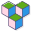CppBuzz# Teradata C & C++ Interview Questions List

It will be Skype of Webex interview where they will share an online editor with to write programs. It was one our discussion on following questions -

Question 1:
Implement memcpy recursively in C/C++ Programming

Question 2:
How to use p to print the value of x in below code snippet

``````
int main()
{
int x=1000;
char *p;
p=&x;
printf("Value of x is %d", *(int *)p );
return 0;
}
``````

Question 3:
Call & Implement swap function to swap pa & pb

``````
main()
{
char a[]="Hello", b[]="World";
char *pa=a, *pb=b;
printf("pa=%s pb=%s\n", pa, pb); //pa=Hello pb=World
swap( );
printf("pa=%s pb=%s\n", pa, pb);//pa=World pb=Hello
printf("a=%s b=%s\n", a, b); //a=Hello b=World
}
void swap()
{

}
``````

Question 4:
Consider the following function S():
S(n) = 1.3 + 3.5 + 5.7 + .. n terms
dot indicates product
Implement S as a recursive function
// 1 3 5 7 9